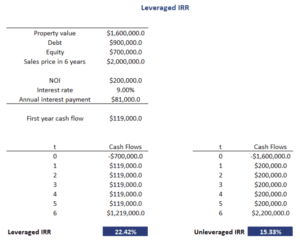# Leveraged IRR Real Estate

The leveraged internal rate of return (IRR) is a measure of the return on investment on a real estate investment when leverage is employed. In most cases, investors will uses leverage when investing in real estate. Thus, it is very important to understand the impact of leverage on an investment. In particular, while leverage increases returns when these returns are positive, returns are also considerably lower when performance is bad.

On this page, we discuss the use of leverage in the context of real estate investments. We calculate both the levered and the unlevered internal rate of return to illustrate the impact of leverage on returns. A spreadsheet that implements both approaches is available at the bottom of the page.

## Implications of leverage

The impact of leverage is twofold. On the one hand, less equity needs to be invested. This increases the return on equity. At the same time, the loan needs to be repaid and interest expense needs to be paid. These repayments are required regardless of performance and therefore reduce cash flows.

## Leveraged IRR example

The best way to calculate the leveraged IRR is by using a financial calculator or by using a spreadsheet. In this case, we will employ a spreadsheet and the IRR function of Excel to calculate the IRR. We will calculate two IRRs; the unleveraged IRR and the leveraged IRR.

The following figure illustrates the calculation of the leveraged IRR and unleveraged IRR for a real estate investment that will be sold again at the end of six years. The loan is an interest-only loan. Thus the full principal is repaid when the property is sold again.## Summary

We discussed how to calculate the unlevered and levered IRR. It is important to stress that leverage will magnify both positive and negative returns. Investors should be aware of the symmetrical impact of using leverage we discussed above.

### Calculator

Want to have an implementation in Excel? Download the Excel file: Leveraged IRR